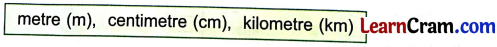# DAV Class 3 Maths Chapter 8 Worksheet 1 Solutions

The DAV Class 3 Maths Solutions and DAV Class 3 Maths Chapter 8 Worksheet 1 Solutions of Length offer comprehensive answers to textbook questions.

## DAV Class 3 Maths Ch 8 WS 1 Solutions

Question 1.
Select the correct unit from the box to measure the following lengths.(a) Length of your skirt/shorts. _________
Solution:
Centimetres (cm)

(b) Height of a room. _________
Solution:
metres (m)

Solution:
centimetres (cm)(d) Distance between Delhi and Agra. _________
Solution:
kilometres (km)

(e) Length of your thumb. _________
Solution:
centimetres (cm)

(f) Distance between the Earth and the Moon. _________
Solution:
kilometres (km)

(g) Length of a canal. _________
Solution:
kilometres (km)

(h) Length of cloth needed to stitch a curtain. _________
Solution:
metres (m)Question 2.
Fill in the blanks.

(a) The biggest unit of length is _________.
Solution:
kilometre

(b) 1 kilometre is equal to _________ metres.
Solution:
1000 metres

(c) The standard unit of length _________.
Solution:
metre (m)

(d) The length of a railway track is measured in _________.
Solution:
kilometre (km)### DAV Class 3 Maths Chapter 8 Worksheet 1 Notes

The standard unit of length is metre.
1 metre = 100 centimetres
In daily life we have some objects which are sold by measuring length.
For example, Ribbon, cloths etc.

Introduction of kilometre

To measure big length and long distances, we make use of the unit called kilometre.
1 kilometre = 1000 metres
kilometre is written as ‘km’
metre is written as ‘m’
centimetre is written as ‘cm’.